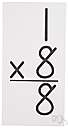# mathematical product

(redirected from Product (mathematics))
Also found in: Thesaurus, Wikipedia.
ThesaurusAntonymsRelated WordsSynonymsLegend:
 Noun 1mathematical product - a quantity obtained by multiplication; "the product of 2 and 3 is 6"productquantity - the concept that something has a magnitude and can be represented in mathematical expressions by a constant or a variablefactorial - the product of all the integers up to and including a given integer; "1, 2, 6, 24, and 120 are factorials"multiple - the product of a quantity by an integer; "36 is a multiple of 9"
Site: Follow: Share:
Open / Close